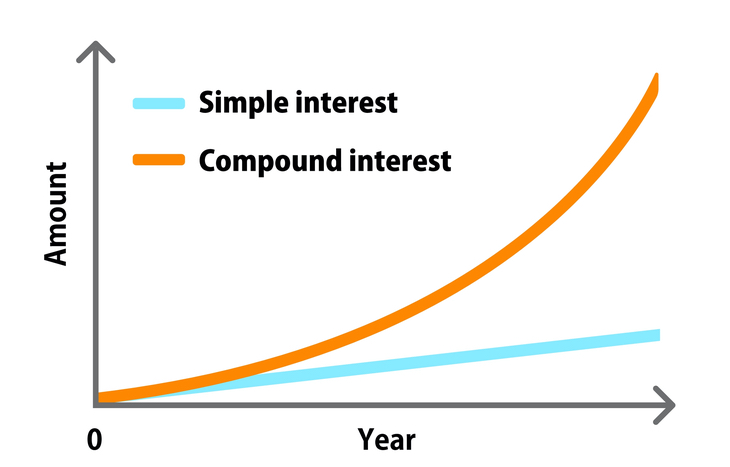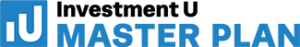Financial Literacy

# Simple Interest vs. Compound Interest

On the surface, an interest rate is just a number. How that number applies to debt or equity opens up a world of possibilities. The first consideration is always whether it’s simple interest vs. compound interest. Depending on which one you’re dealing with, the outcomes for your wealth could be vastly different.

Simple and compound interest are two very different concepts. While they both represent accumulation, the method of accumulation is different. For example, you’d love it if your credit card used simple interest to calculate your balance instead of compound interest! Likewise, you probably don’t want to be too heavily invested in bonds that offer a return calculated by simple interest.

Let’s get to know simple and compound interest: what makes them different and how they apply to investments, both positively and negatively.## What is Simple Interest?

Simple interest is the calculation of the cost of a loan or the total return on a non-compounding principal balance. This means that the principal balance is unchanging—no interest payments compound it over the term of the loan or investment. It’s the most basic form of interest and remains static.

Simple interest tends to benefit borrowers, since it’s non-compounding. Instead of racking up exponential fees over the term of a loan, you’re paying a known amount in interest against the original amount borrowed. As the loan balance goes down each month, so does the interest, since it’s fixed to the outstanding balance.

### Simple Interest Formula

The formula for simple interest is as simple as you might imagine: I = Prt, where:

• I = total interest value
• P = principal value
• r = interest rate
• t = time period

### Simple Interest Examples

• A Certificate of Deposit (CD) will yield an ROI based on the amount of principal invested. The return isn’t compounding, and the interest rate and term are both fixed. If you invest \$10,000 in a 2% CD for one year, your return will be \$200.
• Auto loans amortize monthly, which means you know principal and interest payments in advance. If you buy a car for \$20,000 at a 6% interest rate over 60 months, you’ll owe \$3,200 in interest total. Your monthly payment will remain fixed at ~\$387.

## What is Compound Interest?

Compound interest is an investor’s best friend. This type of interest rolls back into the principal to create a larger balance that’s capable of generating higher returns with each compounding period. The longer the investment and the higher the interest rate, the more wealth generation potential it has.

Compound interest benefits investors with long time horizons. Even if the interest rate fluctuates over time, there’s a longer runway to create exponential growth. It’s also possible to expedite compounding with continued principal investment or through reinvestments, such as dividends. Compounding puts your money to work for you.

### Compound Interest Formula

The formula for compound interest is more complex than simple interest: P(1 +r/n)nt, where:

• P = the initial principal balance
• r = the interest rate
• n = the number of times interest is applied
• t = the number of time periods elapsed

### Compound Interest Examples

• An index fund will create compound growth over time. If you invest \$5,000 and continue to invest another \$250 each month for 40 years at an average interest rate of 8%, your final compounded amount will be almost a million dollars!
• Want to create your own example of compound interest at work? Check out our compound interest calculator to see what happens to an investment when you adjust the principal, time horizon, interest rate and more.

## Pay Attention to Principal

Whether simple or compounded, principal has a huge effect on the power of interest rates. For simple interest rates, the principal will determine the net ROI of your investment or the cost of borrowing money. For compounding investments or debt, the higher the principal, the faster the rate of accumulation.

Investors need to be aware of interest rates no matter which side of the financial transaction they’re on. Consider the relationship between the sum of money in question and the interest rate that accompanies it. In doing so, you’ll have the power to calculate things like ROI or Internal Rate of Return (IRR).

## Know the Best Method for Accumulating Wealth

You’re likely to encounter both types of interest in your time as an investor. The key is knowing which will benefit you more in the context of your investment vehicle: simple interest vs. compound interest. If you’re looking for a low-risk investment in the short-term, you might settle for simple interest. If you’re in it for big gains over a long time period, compound interest is where it’s at.

To expand your investment knowledge further, sign up for the Investment U e-letter below. Our team of experts provide daily investing tips, insights and more.

Your access to simple vs. compound interest will depend on the mode of investment. Good luck finding bonds with compound interest opportunities! Likewise, beware of the compounding power of debt. Take the time to understand the nature of the interest behind the number and how it affects your principal. Then, do everything you can to maximize (or minimize) the effects of interest on your investment.

###### Articles by Leanna Kelly